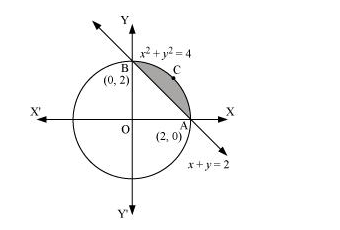# Smaller area enclosed by the circle

Question:

Smaller area enclosed by the circle $x^{2}+y^{2}=4$ and the line $x+y=2$ is

A. $2(\pi-2)$

B. $\pi-2$

C. $2 \pi-1$

D. $2(\pi+2)$

Solution:

The smaller area enclosed by the circle, $x^{2}+y^{2}=4$, and the line, $x+y=2$, is represented by the shaded area ACBA asIt can be observed that,

Area ACBA = Area OACBO – Area (ΔOAB)

$=\int_{0}^{2} \sqrt{4-x^{2}} d x-\int_{0}^{2}(2-x) d x$

$=\left[\frac{x}{2} \sqrt{4-x^{2}}+\frac{4}{2} \sin ^{-1} \frac{x}{2}\right]_{0}^{2}-\left[2 x-\frac{x^{2}}{2}\right]_{0}^{2}$

$=\left[2 \cdot \frac{\pi}{2}\right]-[4-2]$

$=(\pi-2)$ units

Thus, the correct answer is B.